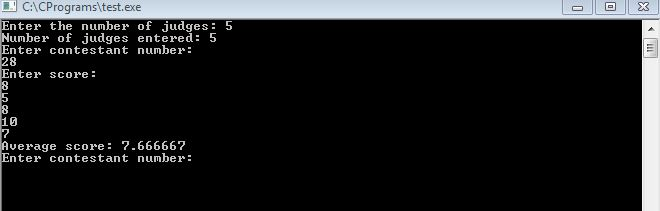# Question & Answer: I need help on this C++ program. I have provided sample output below the program. The variable 'N' must be an int datatype but the findAve…..

I need help on this C++ program. I have provided sample output below the program. The variable ‘N’ must be an int datatype but the findAverageScore( ) function should return a double as shown in the sample output. How can I accomplish this?

/*
EPP Review
Part 1
Gymnastics Competition.
*/

Don't use plagiarized sources. Get Your Custom Essay on
Question & Answer: I need help on this C++ program. I have provided sample output below the program. The variable 'N' must be an int datatype but the findAve…..
GET AN ESSAY WRITTEN FOR YOU FROM AS LOW AS \$13/PAGE

#include <cstdio>
#include <iostream>
#include <iomanip>

using namespace std;

// length is the number of elements in the array
int findAverageScore(int scores[], int length ){
// create variable to store highest and lowest scores
int highest = 0;
int lowest = scores;
int total;

for (int i = 0; i < length; i++) {
total += scores[i];

// Check to see if score is greater than the previous highest score
if (scores[i] >= highest) {
highest = scores[i];
}
// Check to see if score is less than the previous lowest score
if (scores[i] <= lowest) {
lowest = scores[i];
}
}

total = total – highest – lowest;
}

int main(){
int N; //THIS must be an int but averageScore
//should be double.
int maxScore = 0;
int contestant;
int highestContestant;

printf(“Enter the number of judges: “);
cin >> N;
// Array of scores to be stored
int* scores = new int[N];

printf(“Number of judges entered: %dn”, N);

while(true){
puts(“Enter contestant number:”);
cin >> contestant;

if(contestant > 0){

puts(“Enter score: “);
for (int i = 0; i < N; i++) {
cin >> scores[i];
}
int averageScore = findAverageScore(scores, N);
if (averageScore>=maxScore) {
highestContestant = contestant;
maxScore = averageScore;
}
printf(“Average score: %dn”, averageScore);

} else {
printf(“Contestant %d had the highest score. n”, highestContestant);
break;
}
}

return 0;
}

************* SAMPLE OUTPUT *******************

Number of Judges: 5

34 7 5 3 7 6

Contestant 34 6

28 8 5 8 10 7

Contestant 28 7.666666667

3 5 5 3 4 4

Contestant 3 4.333333333

−1

Contestant 28 had the highest score.

For this you need to use datatype as “double” in the function findAverageScore. The modified code is given as below:

#include <cstdio>
#include <iostream>
#include <iomanip>

using namespace std;

// length is the number of elements in the array
double findAverageScore(int scores[], int length ){
// create variable to store highest and lowest scores
double highest = 0;
double lowest = scores;
double total;

for (int i = 0; i < length; i++) {
total += scores[i];

// Check to see if score is greater than the previous highest score
if (scores[i] >= highest) {
highest = scores[i];
}
// Check to see if score is less than the previous lowest score
if (scores[i] <= lowest) {
lowest = scores[i];
}
}

total = total – highest – lowest;
}

int main(){
int N;   //THIS must be an int but averageScore
//should be double.
int maxScore = 0;
int contestant;
int highestContestant;

printf(“Enter the number of judges: “);
cin >> N;
// Array of scores to be stored
int* scores = new int[N];

printf(“Number of judges entered: %dn”, N);

while(true){
puts(“Enter contestant number:”);
cin >> contestant;

if(contestant > 0){

puts(“Enter score: “);
for (int i = 0; i < N; i++) {
cin >> scores[i];
}
double averageScore = findAverageScore(scores, N);
if (averageScore>=maxScore) {
highestContestant = contestant;
maxScore = averageScore;
}
printf(“Average score: %lfn”, averageScore);

} else {
printf(“Contestant %d had the highest score. n”, highestContestant);
break;
}
}

return 0;
}

The Output is shown below: